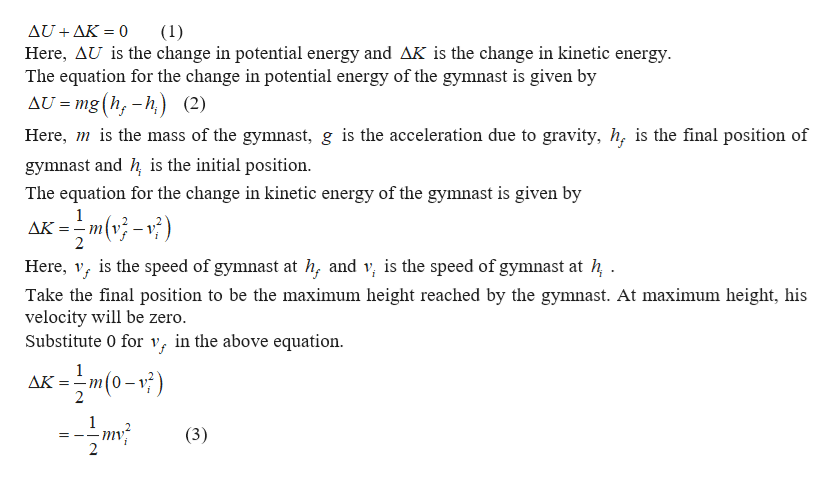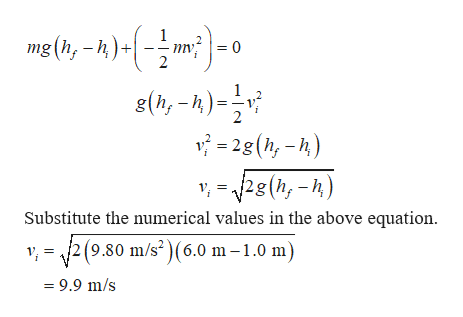# An olympic gymnast jumps up and down on a trampoline. If the gymnast's feet leave the trampoline at a height of 1.0 m above the ground and reach a maximum height of 6.0 m above the ground, at what speed was the gymnast launched from the trampoline?

Question
20 views

An olympic gymnast jumps up and down on a trampoline. If the gymnast's feet leave the trampoline at a height of 1.0 m above the ground and reach a maximum height of 6.0 m above the ground, at what speed was the gymnast launched from the trampoline?

check_circle

Step 1

According to the principle of conservation of energy, the sum of the change in potential energy of the gymnast and the change in his kinetic energy will be equal to zero.help_outlineImage TranscriptioncloseAUAK 0 Here, AU is the change in potential energy and AK is the change in kinetic energy The equation for the change in potential energy of the gymnast is given by (1) AU mg (h,-h) (2) Here, is the mass of the gymnast, g is the acceleration due to gravity, h, is the final position of gymnast and h is the initial position The equation for the change in kinetic energy of the gymnast is given by 1 AK m-) is the speed of gymnast at h, and v, is the speed of gymnast at h Here, Take the final position to be the maximum height reached by the gymnast. At maximum height, his velocity will be zero. Substitute 0 for v, in the above equation xm(0-) AK mv (3) fullscreen
Step 2

Put equations (2) and (3)...help_outlineImage Transcriptionclosemg (h,-h) =0 s(,-) 2 - 2g (h -) ) ,2g(h,-h Substitute the numerical values in the above equation. 2(9.80 m/s2)(6.0 m -1.0 m) 9.9 m/s fullscreen

### Want to see the full answer?

See Solution

#### Want to see this answer and more?

Solutions are written by subject experts who are available 24/7. Questions are typically answered within 1 hour.*

See Solution
*Response times may vary by subject and question.
Tagged in
SciencePhysics

### Newtons Laws of Motion